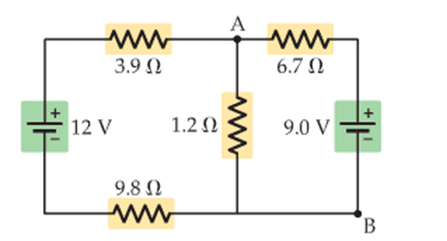# Determine the difference in potential between A and B

In summary, Kirchhoff's voltage law can be used to find the potential difference between two points if there is a continuous path between them.f

## Homework Statement

Determine the difference in potential between A and B.## Homework Equations

Kirchhoff's rules for current (loop, junction), V = IR

## The Attempt at a Solution

I have found the currents through the resistors:
12 - 3.9a - 1.2b - 9.8a = 0 (loop rule)
12 - 3.9a - 6.7c - 9 - 9.8a = 0 (loop rule)
a = b + c (junction rule)
c = -1.0295 & b = 1.75196 & a = 0.722456

6.7 ohm resistor has 1.02 A counterclockwise
3.9 ohm and 9.8 ohm resistors have 0.72 A clockwise
1.2 ohm resistor has 1.7 A clockwise.

What do I do from here? I know that if A and B were in series, the difference in potential between them would be a drop which equals the potential differences across each component between them in series, but this is in parallel, and so I don't know what to do.

Kirchhoff's voltage law works for any components along a continuous path. If you can trace a path from B to A and can add up all the voltage changes along the way, you're done!

There is a 1.2 ohm resistor on one path between A and B, and the current through it is 1.7 A, so the potential difference across the resistor is 2.04 V. Is this the difference in potential between A and B? The correct answer is 2.2 V.

There is a 1.2 ohm resistor on one path between A and B, and the current through it is 1.7 A, so the potential difference across the resistor is 2.04 V. Is this the difference in potential between A and B? The correct answer is 2.2 V.

If you keep a few more decimal places in your intermediate values you should find that the voltage is a bit higher than what you got (although not quite 2.2 V).

You could also try the same thing for other paths between A and B.

1.2 ohms * 1.75196 A = 2.102352 V

Still coming up short.Using the other available path,
-6.7*1.0295 + 9 = 2.102352 V

Hmm... I suppose it's close enough.

1.2 ohms * 1.75196 A = 2.102352 V

Still coming up short.Using the other available path,
-6.7*1.0295 + 9 = 2.102352 V

Hmm... I suppose it's close enough.

Not only is it close enough, 2.1V is the correct answer! Sometimes books can be, shall we say, not entirely correct.

Got it, thanks for your help.